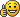## Changing the speed of a circular motion behaves strangely

### Changing the speed of a circular motion behaves strangely

Hi,

To move an object in a circle over time we can convert polar to cartesian coordinates by using parametric equation

x = x + radius * sin(time * speed)
y = y + radius * cos(time * speed)

In JS or other language changing speed changes only velocity of the object, radius stays the same.
In Algodoo changing speed parameter changes not only velocity of the object but also its radius.
How can I make it work like it should.

Code: Select all
`(e)=>{    r = 0.5;    speed = 5;    a = (sim.time * speed);    pos = pos + r * [sin(a), cos(a)]}`

Thanks,
algouser

Posts: 2
Joined: Sat Mar 16, 2019 1:07 pm

### Re: Changing the speed of a circular motion behaves strangely

You'r error is how you apply the circle motion. Instead of SETTING the position to the circle-coordinates(pos = [cos,sin]), you are ADDING it, thus having a kind of circle VELOCITY instead of truly following a circle. Changing the speed at which the velocity turns effectively increases the curvature of the circle, and thus decreases the radius unless you increase the magnitude of the velocity itself. The correct script that does what you want looks like this:

Rigid circle movement:
r = 1;
f = 1; (Motion frequency. Higher numbers mean more revolutions per second);
p = [1,3]; (center coordinates)
t = sim.frequency*2*math.pi*f;
pos = p+r*[math.cos(t),math.sin(t)];

Circular velocity, allowing for you to move the object without having to change a variable:

r = 1;
f = 1;
v = 2 * math.pi * f;
t = sim.time * 2 * math.pi * f;
d = [math.cos(t), math.sin(t)];
vel = v * d

note: changing frequency results in the center of the circle changing aswell(but not the radius), to prevent this you would need to keep track of the "circle progress"

(type this in the empty, unlabelled black textbox in the top left of the script menu)
_rev = 0

r = 1;
f = 1;
_rev = _rev + f * e.dt;
t = _rev * 2 * math.pi;
d = [math.cos(t), math.sin(t)];
vel = d * f * r * (2 * math.pi)
FRA32

Posts: 213
Joined: Wed Dec 03, 2014 9:51 pm

### Re: Changing the speed of a circular motion behaves strangely

I'm moving an object in a circular motion at a fluctuating velocity at given fix radius.
first and last codes work
Thank Youalgouser

Posts: 2
Joined: Sat Mar 16, 2019 1:07 pm

### Re: Changing the speed of a circular motion behaves strangely

I literally understood nothing that was said.Hello my name is Kenlimepie. I'm an Algodoo Enthusiast, and Marble Racer. I run a Youtube channel which is about Algodoo Marble Racing, but I am not afraid to design new ideas in this program. https://www.youtube.com/c/kenlimepieKen3344

Posts: 53
Joined: Mon Sep 05, 2016 8:44 pm